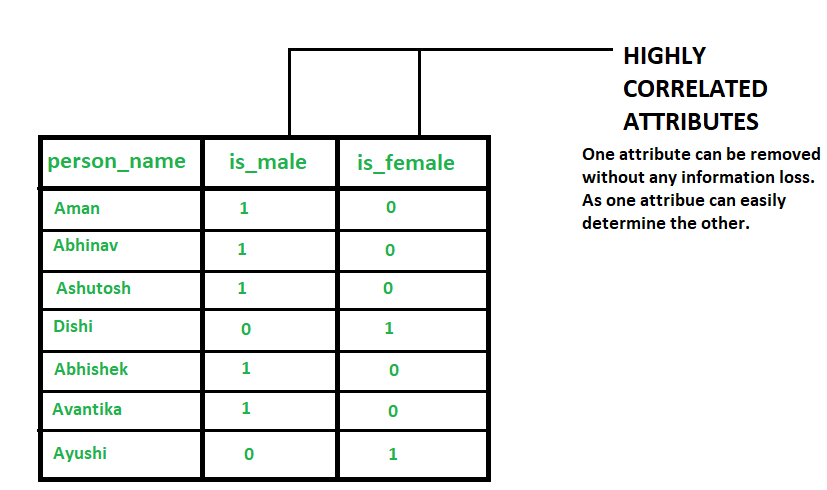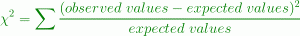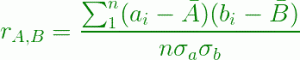# Redundancy and Correlation in Data Mining

Prerequisites:Chi-square test, covariance-and-correlation

### What is Data Redundancy ?

During data integration in data mining, various data stores are used. This can lead to the problem of redundancy in data. An attribute (column or feature of data set) is called redundant if it can be derived from any other attribute or set of attributes. Inconsistencies in attribute or dimension naming can also lead to the redundancies in data set.

Example –
We have a data set having three attributes- `person_name`, `is_male`, `is_female`.

• `is_male` is 1 if the corresponding person is a male else it is 0 .
• `is_female` is 1 if the corresponding person is a female else it is 0.
•On analysing the fact that if a person is not male (i.e `is_male` is 0 corresponding the person_name) then, the person is surely a female (since there are only two value in output class- male and female). It implies that the two attributes are highly correlated and one attribute can determine the other. Hence, one of these attributes became redundant. So one of these two attributes can be dropped without any information loss.

### Detection of Data Redundancy –

Redundancies can be detected using following methods

• χ2Test (Used for nominal Data or categorical or qualitative data)
• Correlation coefficient and covariance (Used for numeric Data or quantitative data)
• χ2 Test for Nominal Data –
This test is performed over nominal data.Let there are two attributes A and B in a data set.A contingency table is made for representing data tuples.
The formula used for this test is:Where observed values are the actual count and expected values are the count obtained from contingency table joint events.

The χ2 checks the hypothesis that A and B are independent. If this hypothesis can be rejected, we can say that A and B are statistically correlated and one of them (either A or B) can be discarded.

### Correlation Coefficient for Numeric Data –

This test is used for numeric data.In this case the correlation between attributes(say A and B) is computed by Pearson’s product moment coefficient also known as correlation coefficient
Formula used is:Where n is the number of tuples, ai, bi are the respective values of A and B in tuple i.

Conclusion: Higher the correlation coefficient, more strongly the attributes are correlated and one of them (either A or B) can be discarded. If the correlation constant is 0 then the attributes are independent and if it is negative then one attribute discourages the other i.e if value of one attribute increases then value of other decreases.

My Personal Notes arrow_drop_upCheck out this Author's contributed articles.

If you like GeeksforGeeks and would like to contribute, you can also write an article using contribute.geeksforgeeks.org or mail your article to contribute@geeksforgeeks.org. See your article appearing on the GeeksforGeeks main page and help other Geeks.

Please Improve this article if you find anything incorrect by clicking on the "Improve Article" button below.

Article Tags :
Practice Tags :

Be the First to upvote.

Please write to us at contribute@geeksforgeeks.org to report any issue with the above content.